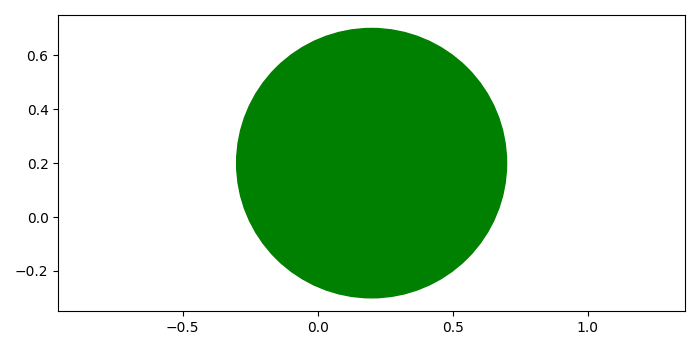# How to plot a circle in Matplotlib?

To plot a circle in matplotlib, we can take the following steps −

• Create a new figure or activate an existing figure using figure() method.

• Add a subplot arrangement to the current axis.

• Create a true circle at a center using Circle class.

• Add a patch to the current axis.

• Set limits of the x and y axes.

• To display the figure, use show() method.

## Example

from matplotlib import pyplot as plt, patches
plt.rcParams["figure.figsize"] = [7.00, 3.50]
plt.rcParams["figure.autolayout"] = True
fig = plt.figure()
plt.show()